# Question 8 value 9p Show that the curve ที่(t-(2 + V2 cost, 1-sint, 3 + sin t , t e R lies at the...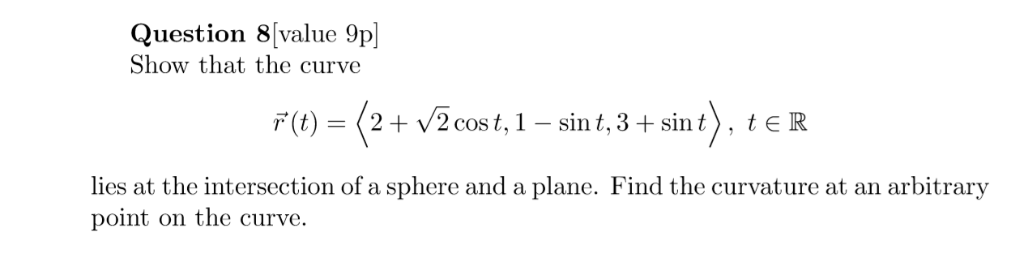Question 8 value 9p Show that the curve ที่(t-(2 + V2 cost, 1-sint, 3 + sin t , t e R lies at the intersection of a sphere and a plane. Find the curvature at an arbitrary point on the curve.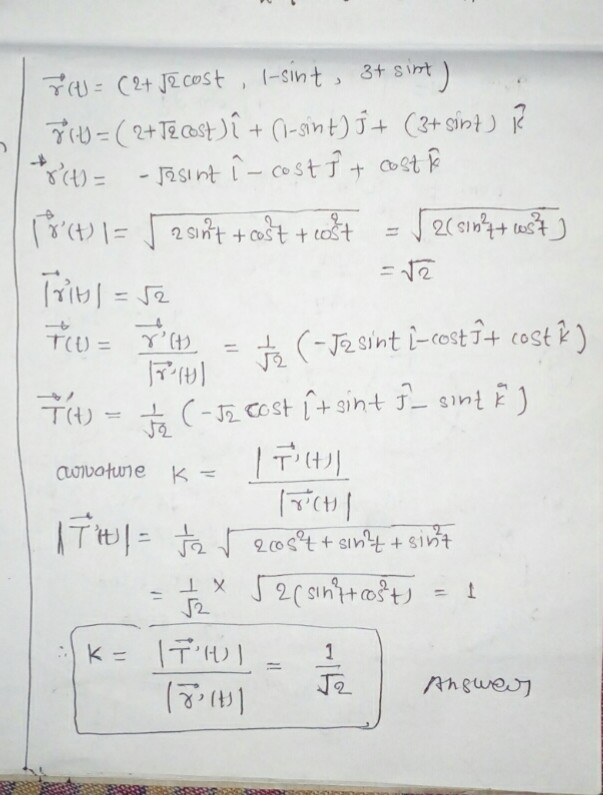#### Earn Coin

Coins can be redeemed for fabulous gifts.

Similar Homework Help Questions
• ### Question 1. Let y : R -> R' be the parametrised curve 8 (t)= 1+ sin...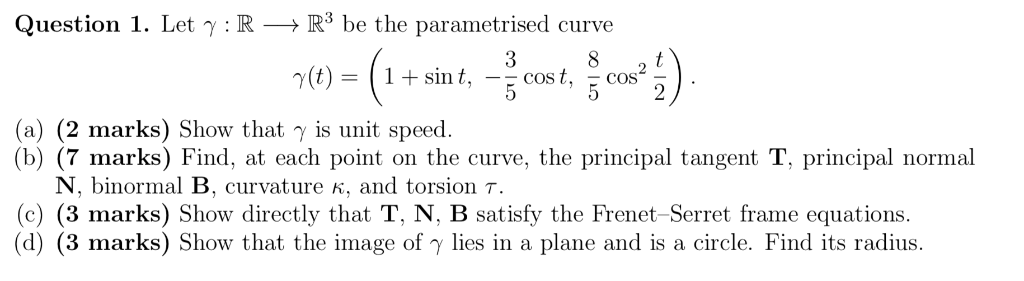Question 1. Let y : R -> R' be the parametrised curve 8 (t)= 1+ sin t Cost 5 Cos (a) (2 marks) Show that y is unit speed (7 marks) Find, at each point on the curve, the principal tangent T, principal normal (b) N, binormal B, curvature K, and torsion 7. (c) (3 marks) Show directly that T, N, B satisfy the Frenet-Serret frame equations (d) (3 marks) Show that the image of y lies in a plane...

• ### 3. Let C be the curve r(t) = < sint, cost, t>,0 sts 1/2. Evaluate the...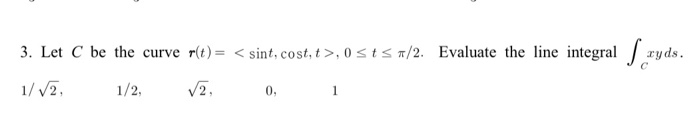3. Let C be the curve r(t) = < sint, cost, t>,0 sts 1/2. Evaluate the line integral S ry ryds. 1/V2. 1/2, V2, 0,

• ### X) 13.4.21 Find an equation for the circle of curvature of the curve r(t)-21 + sin(t) j at the point (z,1). (The curve...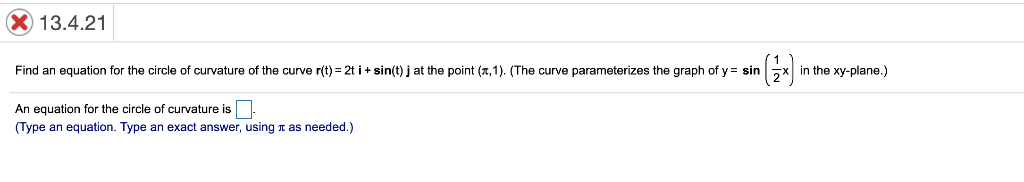X) 13.4.21 Find an equation for the circle of curvature of the curve r(t)-21 + sin(t) j at the point (z,1). (The curve parameterizes the graph of y = sin | 2x | in the xy-plane.) An equation for the circle of curvature is (Type an equation. Type an exact answer, using π as needed.) X) 13.4.21 Find an equation for the circle of curvature of the curve r(t)-21 + sin(t) j at the point (z,1). (The curve parameterizes the...

• ### 3. (12 points) Consider the curve C defined by r(t) = (4 sint, -4 cost,0) with...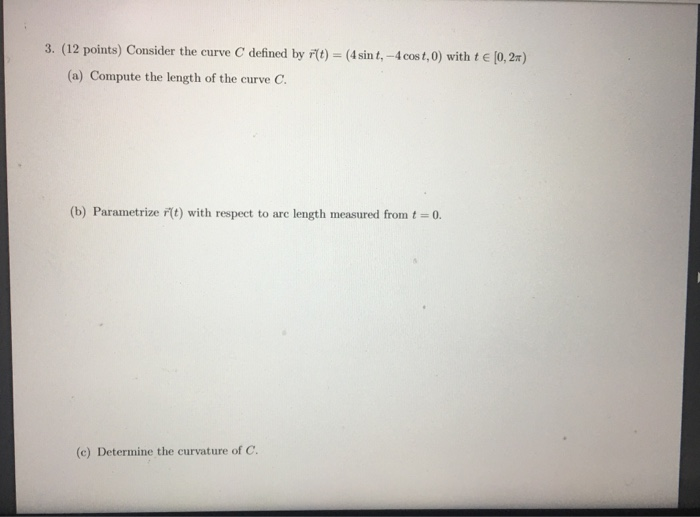3. (12 points) Consider the curve C defined by r(t) = (4 sint, -4 cost,0) with t€ (0,2) (a) Compute the length of the curve C. (b) Parametrize fit) with respect to are length measured from t = 0. (c) Determine the curvature of C.

• ### 34. Let r(t) e' cos(2nt), e' sin(2nt) >, for t 2 0. a) Sketch the plane...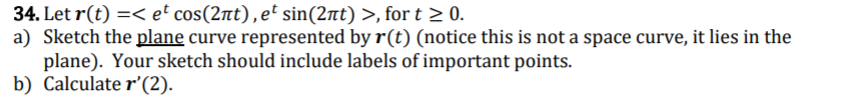34. Let r(t) e' cos(2nt), e' sin(2nt) >, for t 2 0. a) Sketch the plane curve represented by r(t) (notice this is not a space curve, it lies in the plane). Your sketch should include labels of important points b) Calculate r'(2) 34. Let r(t) e' cos(2nt), e' sin(2nt) >, for t 2 0. a) Sketch the plane curve represented by r(t) (notice this is not a space curve, it lies in the plane). Your sketch should include labels...

• ### (22 - y2 + 2)ds, here C is the curve r(t) = (3 cost, 3 sint,...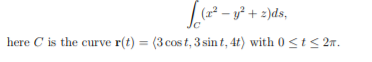(22 - y2 + 2)ds, here C is the curve r(t) = (3 cost, 3 sint, 4t) with 0 <t<2.

• ### At least one of the answers above is NOT correct. (1 point) For the curve given...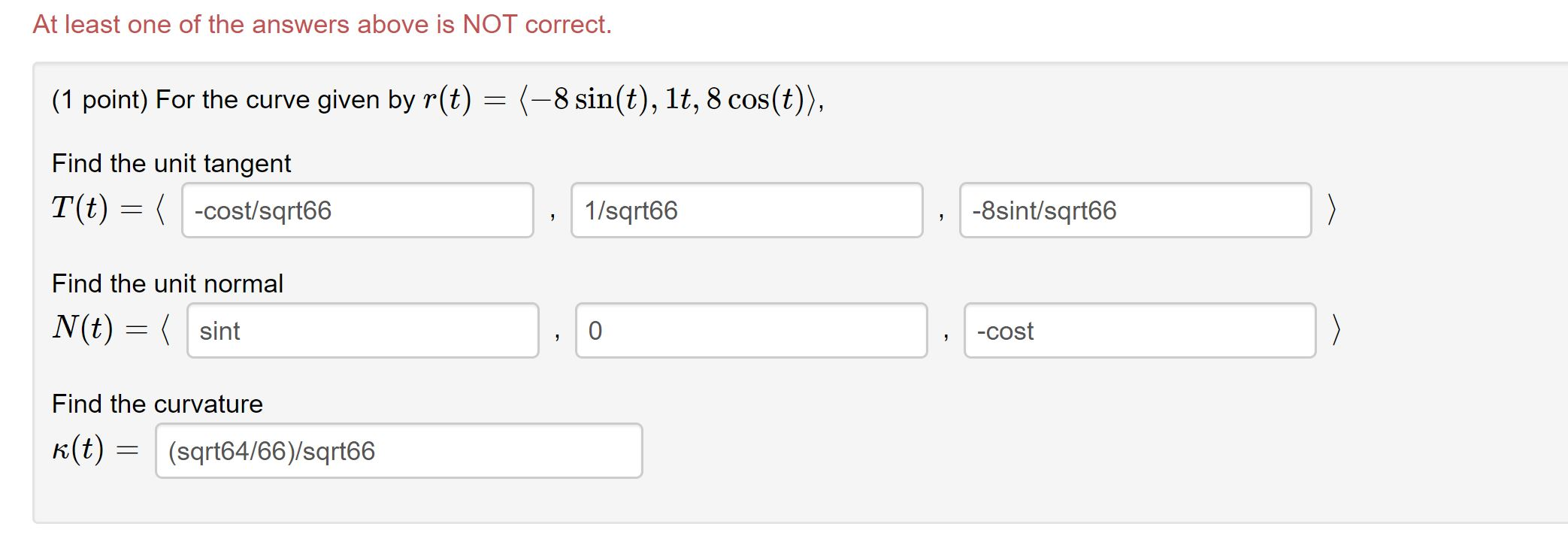At least one of the answers above is NOT correct. (1 point) For the curve given by r(t) = (-8 sin(t), 1t, 8 cos(t)), Find the unit tangent T(t) = -cost/sqrt66 1/sqrt66 -8 sint/sqrt66 > Find the unit normal N(t) = ( sint 0 -cost Find the curvature k(t) = (sqrt64/66)/sqrt66

• ### Find the curvature of the curve r(t) = (3 cos(4t), 3 sin(4t), t) at the point...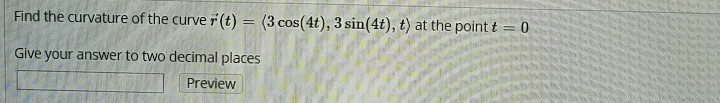Find the curvature of the curve r(t) = (3 cos(4t), 3 sin(4t), t) at the point t = 0 Give your answer to two decimal places Preview

• ### Find the point of intersection of the tangent lines to the curve r(t) = 3 sin(πt),...

Find the point of intersection of the tangent lines to the curve r(t) = 3 sin(πt), 4 sin(πt), 6 cos(πt) at the points where t = 0 and t = 0.5.

• ### 2. Find the volume of the solid that lies between the paraboloidand the plane -2x. Show your work...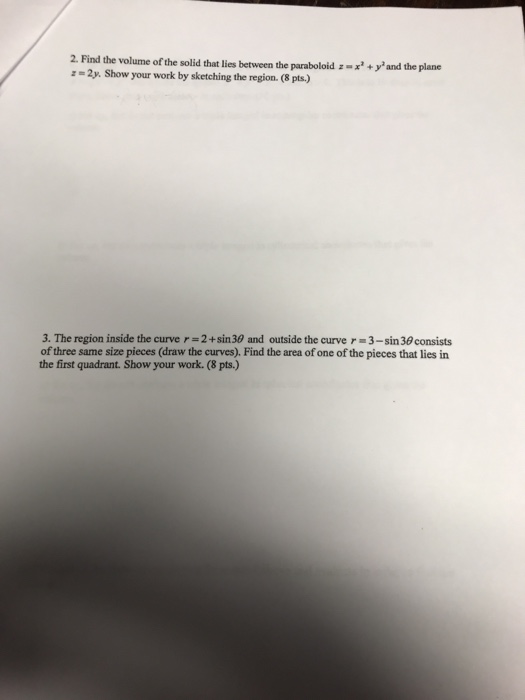please answer both questions 2. Find the volume of the solid that lies between the paraboloidand the plane -2x. Show your work by sketching the region. (8 pts.) 3. The region inside the curve r-2+sin 30 and outside the curve r 3-sin 30 consists of three same size pieces (draw the curves). Find the area of one of the pieces that lies in the first quadrant. Show your work. (8 pts.) 2. Find the volume of the solid that lies...

Free Homework App